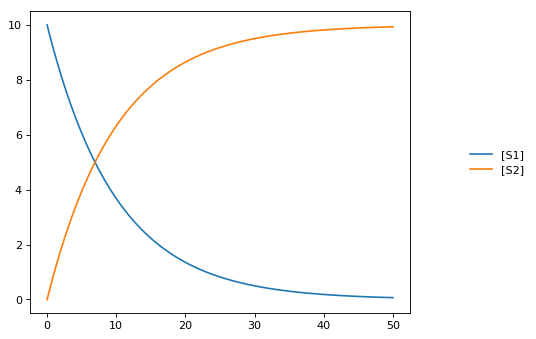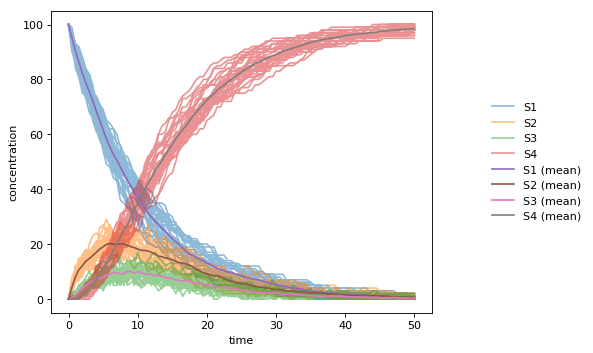Quick Start¶

To get started using Tellurium, download one of the front-ends. Then, follow the examples below to get an idea of how to load and simulate models.

Simple Example¶

This shows how to set up a simple model in Tellurium and solve it as an ODE. Tellurium uses a human-readable representation of SBML models called Antimony. The Antimony code for this example contains a single reaction with associated kinetics. After creating the Antimony string, use the loada function to load it into the RoadRunner simulator. A RoadRunner instance has a method simulate that can be used to run timecourse simulations of a model, as shown below.

import tellurium as te
r = te.loada('S1 -> S2; k1*S1; k1 = 0.1; S1 = 10')
r.simulate(0, 50, 100)
r.plot()More Complex Example¶

Tellurium can also handle stochastic models. This example shows how to select Tellurium’s stochastic solver. The underlying simulation engine used by Tellurium implements a Gibson direct method for simulating this model.

import tellurium as te
import numpy as np

J1: S1 -> S2;  k1*S1;
J2: S2 -> S3; k2*S2 - k3*S3
# J2_1: S2 -> S3; k2*S2
# J2_2: S3 -> S2; k3*S3;
J3: S3 -> S4; k4*S3;

k1 = 0.1; k2 = 0.5; k3 = 0.5; k4 = 0.5;
S1 = 100;
''')

# use a stochastic solver
r.integrator = 'gillespie'
r.integrator.seed = 1234
# selections specifies the output variables in a simulation
selections = ['time'] + r.getBoundarySpeciesIds() + r.getFloatingSpeciesIds()
r.integrator.variable_step_size = False

# run repeated simulation
Ncol = len(r.selections)
Nsim = 30
points = 101
s_sum = np.zeros(shape=[points, Ncol])
for k in range(Nsim):
r.resetToOrigin()
s = r.simulate(0, 50, points, selections=selections)
s_sum += s
# use show=False to add traces to the current plot
# instead of starting a new one, equivalent to MATLAB hold on
r.plot(s, alpha=0.5, show=False)

# add mean curve, legend, show everything and set labels, titels, ...
fig = te.plot(s[:,0], s_sum[:,1:]/Nsim, names=[x + ' (mean)' for x in selections[1:]], title="Stochastic simulation", xtitle="time", ytitle="concentration")